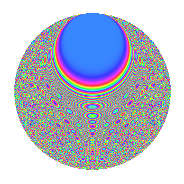# Properties

 Label 1575.2.coLevel 1575 Weight 2 Character orbit co Rep. character $$\chi_{1575}(157,\cdot)$$ Character field $$\Q(\zeta_{12})$$ Dimension 560 Sturm bound 480

# Related objects

## Defining parameters

 Level: $$N$$ = $$1575 = 3^{2} \cdot 5^{2} \cdot 7$$ Weight: $$k$$ = $$2$$ Character orbit: $$[\chi]$$ = 1575.co (of order $$12$$ and degree $$4$$) Character conductor: $$\operatorname{cond}(\chi)$$ = $$315$$ Character field: $$\Q(\zeta_{12})$$ Sturm bound: $$480$$

## Dimensions

The following table gives the dimensions of various subspaces of $$M_{2}(1575, [\chi])$$.

Total New Old
Modular forms 1008 592 416
Cusp forms 912 560 352
Eisenstein series 96 32 64

## Trace form

 $$560q - 2q^{2} + 6q^{3} + 2q^{7} + 8q^{8} + O(q^{10})$$ $$560q - 2q^{2} + 6q^{3} + 2q^{7} + 8q^{8} - 24q^{11} + 6q^{12} + 260q^{16} + 30q^{17} + 26q^{18} + 48q^{21} + 12q^{22} - 12q^{23} - 24q^{26} - 36q^{27} - 4q^{28} - 12q^{31} - 30q^{32} - 24q^{33} - 80q^{36} + 4q^{37} + 24q^{41} + 10q^{42} + 4q^{43} + 16q^{46} + 6q^{47} + 18q^{48} + 44q^{51} - 16q^{53} + 68q^{56} - 8q^{57} + 44q^{58} + 60q^{61} - 54q^{63} - 84q^{66} - 2q^{67} + 32q^{71} - 10q^{72} + 12q^{73} - 48q^{76} + 106q^{77} + 62q^{78} - 52q^{81} + 24q^{82} - 84q^{83} - 184q^{86} + 24q^{87} + 84q^{88} - 16q^{91} + 8q^{92} - 28q^{93} - 180q^{96} - 120q^{98} + O(q^{100})$$

## Decomposition of $$S_{2}^{\mathrm{new}}(1575, [\chi])$$ into newform subspaces

The newforms in this space have not yet been added to the LMFDB.

## Decomposition of $$S_{2}^{\mathrm{old}}(1575, [\chi])$$ into lower level spaces

$$S_{2}^{\mathrm{old}}(1575, [\chi]) \cong$$ $$S_{2}^{\mathrm{new}}(315, [\chi])$$$$^{\oplus 2}$$

## Hecke Characteristic Polynomials

There are no characteristic polynomials of Hecke operators in the database